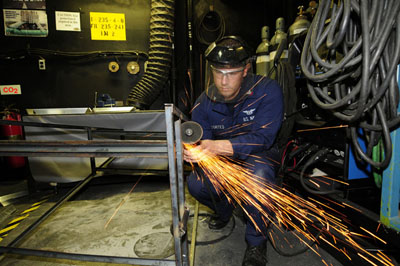## Conservation of Energy in Rotational Motion

Energy is conserved in rotational motion just as in translational motion.

### Learning Objectives

Conclude the interchangeability of force and radius with torque and angle of rotation in determining force

### Key Takeaways

#### Key Points

• Rotating objects have rotational kinetic energy.
• Rotational kinetic energy can change form if work is done on the object.
• Energy is never destroyed, if rotational energy is gained or lost, something must have done work on it to change the form of the energy.

#### Key Terms

• work: A measure of energy expended in moving an object; most commonly, force times displacement. No work is done if the object does not move.
• angular velocity: A vector quantity describing an object in circular motion; its magnitude is equal to the speed of the particle and the direction is perpendicular to the plane of its circular motion.
• rotational inertia: The tendency of a rotating object to remain rotating unless a torque is applied to it.

In this atom we will discuss work and energy associated with rotational motion. shows a worker using an electric grindstone propelled by a motor. Sparks are flying, and noise and vibration are created as layers of steel are pared from the pole. The stone continues to turn even after the motor is turned off, but it is eventually brought to a stop by friction. Clearly, the motor had to work to get the stone spinning. This work went into heat, light, sound, vibration, and considerable rotational kinetic energy.Grindstone: The motor works in spinning the grindstone, giving it rotational kinetic energy. That energy is then converted to heat, light, sound, and vibration. (Credit: U.S. Navy photo by Mass Communication Specialist Seaman Zachary David Bell. )

Work must be done to rotate objects such as grindstones or merry-go-rounds. The simplest rotational situation is one in which the net force is exerted perpendicular to the radius of a disc and remains perpendicular as the disc starts to rotate. The force is parallel to the displacement, and so the net work (W) done is the product of the force (F) and the radius (r) of the disc (this is otherwise known as torque(τ)) times the angle (θ) of rotation:

$\text{W}=\text{Fr}\theta=\tau \theta$.

Work and energy in rotational motion are completely analogous to work and energy in translational motion and completely transferrable. Just as in translational motion (where kinetic energy equals 1/2mv2 where m is mass and v is velocity ), energy is conserved in rotational motion. Kinetic energy (K.E.) in rotational motion is related to moment of rotational inertia (I) and angular velocity (ω):

$\text{KE}=\frac {1}{2}\text{I}\omega ^2$.

The final rotational kinetic energy equals the work done by the torque:

$\text{W}=\tau \theta = \frac {1}{2} \text{I} \omega ^2 =\text{KE}$.

This confirms that the work done went into rotational kinetic energy. To return to the grindstone example, work was done to give the grindstone rotational energy, and work is done by friction so that it loses kinetic energy. However, the energy is never destroyed; it merely changes form from rotation of the grindstone to heat when friction is applied.